Updated date:

# Short-Run Average and Marginal Cost Curves

## Meaning of Short-Run

In the short-run, some factors are fixed while others are variable. The fixed factors are plant, equipment and a unique kind of skilled labor. Short-run is defined as that period in which the firm can expand or contract its output only by varying the amounts of variable factors such as labor and raw materials. In the short period, the size of the plant cannot be altered. More production is possible only by over working the existing plant or by hiring more workers and by purchasing and using more raw materials.

Short-Run Fixed and Variable Costs

Fixed costs are those costs that are invariant to the rate of production. They are the costs of indivisible factors such as building, machinery, vehicles etc. Once these costs are incurred, they can be used over a period at no further cost. Even if production is nil, fixed cost will have to remain the same.

Fixed costs usually include the following:

1. Rent for building

2. Interest for capital

3. Insurance premium

4. Property, business taxes etc.

5. Depreciation

6. Salaries of permanent staff

Variable costs vary with the level of output. It rises when output expands and falls when output contracts. When output is nil, variable cost becomes zero.

Variable costs usually include the following:

1. Payments for raw materials

2. Wages for labor

3. Fuel and power charges

4. Excise duties, sales tax etc.

5. Interest on short term loans

6. Transport costs

## Table 1: Cost Schedule

Total Output Units (1)Total Fixed Cost (2)Total Variable Cost (3)Total Cost (4) - (2 + 3)Average Fixed Cost (5) - (2/1)Average Variable Cost (6) - (3/1)Average Cost (7) - (4/1)Marginal Cost (8)

0

50

0

50

--

--

--

--

1

50

50

100

50

50

100

50

2

50

78

128

25

39

64

28

3

50

98

148

16.7

32.7

49.3

20

4

50

112

162

12.5

28

40.5

14

5

50

130

180

10

26

36

18

6

50

150

200

8.3

25

33.5

20

7

50

175

225

7.1

25

32.1

25

8

50

204

254

6.3

25.5

31.8

29

9

50

242

292

5.6

26.9

32.4

38

10

50

300

350

5

30

35

58

11

50

385

435

4.5

35

39.5

85

Total Fixed Cost

The total obligations of the firm per time for all fixed inputs are called total fixed cost. Total fixed cost remains ‘constant’ or ‘fixed’ irrespective of the size of the output. Suppose Mr. Marcel has borrowed a certain sum of money from Tino to produce knives. Every month he has to pay \$200 towards interest charges. For some reason or other, the business unit is temporarily closed for a few months. Now production is zero, yet he has to pay the interest at the fixed stipulated rate.

Again as production starts and goes on increasing, the total fixed cost remains the same. Diagrammatically the total cost curve is a straight line parallel to the OX-axis.

Total Variable Cost

Total variable cost varies with output. In the above example of Marcel, when the production was temporarily shut down, the variable cost was zero. As output increases, the total variable cost also increases. However, the variation in variable cost is not in proportion to the variation in output. Production is subject to the laws of increasing, constant and diminishing returns. Up to 4 units, the total variable cost increases, but at a diminishing rate. During the intermediary stage of 5 and 6 units variable cost increases but at a diminishing rate. After 6 units of output, total variable cost increases at an increasing rate. Diagrammatically the total variable cost curve is an upward sloping curve.

Total Cost

Total cost is the sum of total fixed cost and total variable cost.

TC = TFC + TVC

Where,

TC= Total Cost

TFC = Total Fixed Cost

TVC = Total Variable Cost

However, it should be noted that total fixed cost is the same irrespective of the level of output. Therefore, a change in total cost is influenced by the change in variable cost only.

The relationship between total fixed cost, total variable cost and total cost will be clear from the following diagram. (figure 1)

Average Fixed Cost

The average fixed cost is the fixed cost per unit of output. It is obtained by dividing the total cost by the number of units of the commodity produced.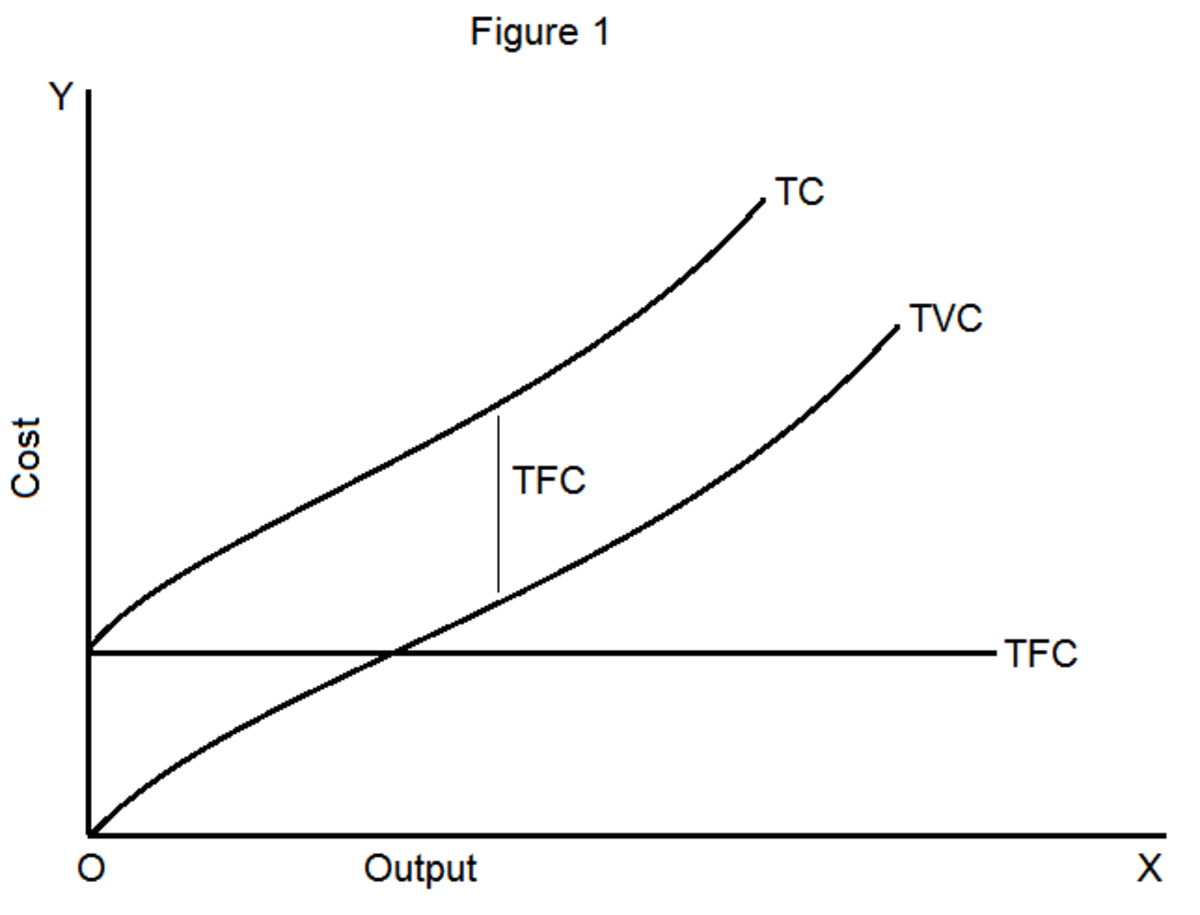Symbolically AFC = TFC/Q

Or AFC = AC – AVC

The average fixed cost (AFC) is a rectangular hyperbola, which never meets the horizontal or vertical axis. Since the total fixed cost is constant, as total output becomes larger and larger, the entire AFC must become smaller and smaller. This continuous falling of AFC, as output expands, is what business people commonly call ‘spreading the overhead.’

Average Variable Cost

The average variable cost is the variable cost per unit of output. It is obtained by dividing the total variable cost by the number of units of the commodity produced.

Symbolically AVC = TVC/Q

Or AVC = AC – AFC

Diagrammatically, the AVC is ‘U’ shaped. The law of variable proportions provides the fundamental explanation for the shape of this curve. It means that the AVC curve first falls, reaches a minimum and then begins to increase.

Average Total Cost or Average Cost

Average cost or average cost is the cost per unit of output. It is obtained by dividing the total cost by the total number of units of the commodity produced.

Symbolically, ATC = TC/Q

TC = TFC + TVC

Therefore, ATC = (TFC+TVC)/Q = (TFC/Q) + (TVC/Q)

ATC = AFC + AVC

The AC curve ‘U’ shaped in nature.

Why the Short-run Average Cost Curve is ‘U’ Shaped?

Prof. Stonier and Hague have given a simple and advanced explanation for the ‘U’ shaped average cost curve.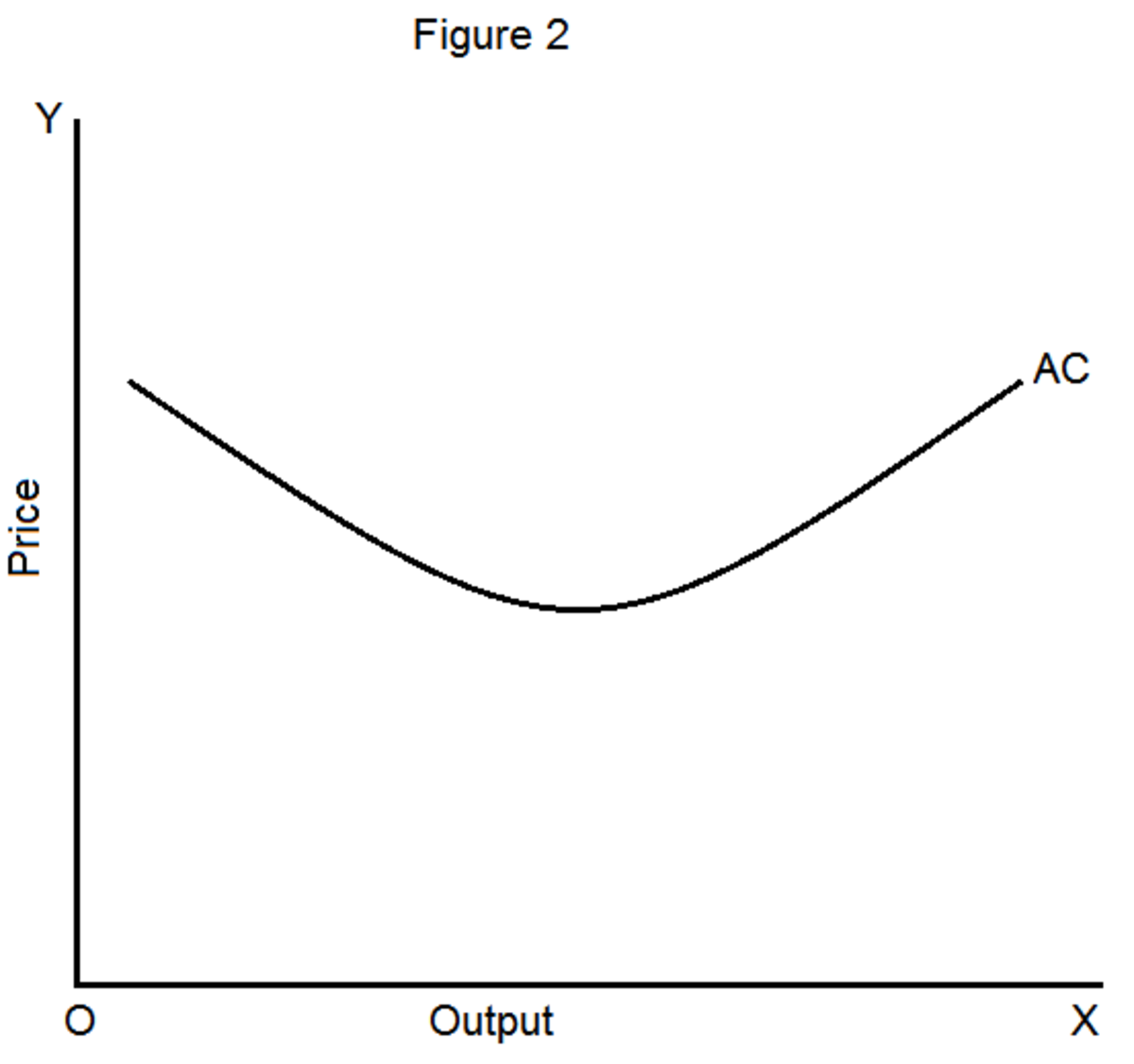Simple Explanation

The average cost is the sum of AFC and AVC. In the initial stages, both the AFC and AVC go on falling. Consequently, the AC also goes on falling. After a certain stage, the AVC starts rising. The AFC falls no doubt but the rise in AVC is greater than the fall in the AFC. Therefore, the AC goes on rising after reaching a minimum point.

Advanced Explanation

When the size of a firm expands, there emerges certain economies of scale. The economies of scale reduce the average cost of production. The important economies are:

1. Technical economies

Technical economies arise because of the adoption of new and scientifically developed machinery.

2. Managerial economies

The unit cost of administration falls as production expands.

3. Marketing economies

The average cost of marketing tends to be lower when a larger output is involved.

4. Financial economies

As the size of the firm expands, it can borrow funds at lower rates of interest.

4. Risk-bearing economies

As a firm expands its output, the statistical law of large numbers works out in its favor. It can diffuse the risk on various products and in different channels of production.

Therefore, the operation of the law of increasing returns helps to reduce the average cost of production. However, after a certain limit due to the emergence of certain diseconomies, the AC curve starts rising. Thus, the average cost curve is ‘U’ shaped.

Marginal Cost

Marginal cost is an addition to the total cost caused by producing one more unit of output.

Marginal cost = change in total cost/change in output

Or MC = ΔTC/ΔQ; where ΔTC is change in total cost, ΔQ is change in output.

It is worth noting that MC is independent of output. It is only the variable cost that varies with a variation in output in the short-run. Therefore, the marginal costs are in fact due to the changes in variable costs. This can be proved algebraically as:

MC = - TCn-1 = (TVCn + TFC) – (TVCn-1 + TFC) = (TVCn – TVCn-1) + (TFC – TFC) = TVCn – TVCn-1

The various cost curves can be shown as shown in the following diagram.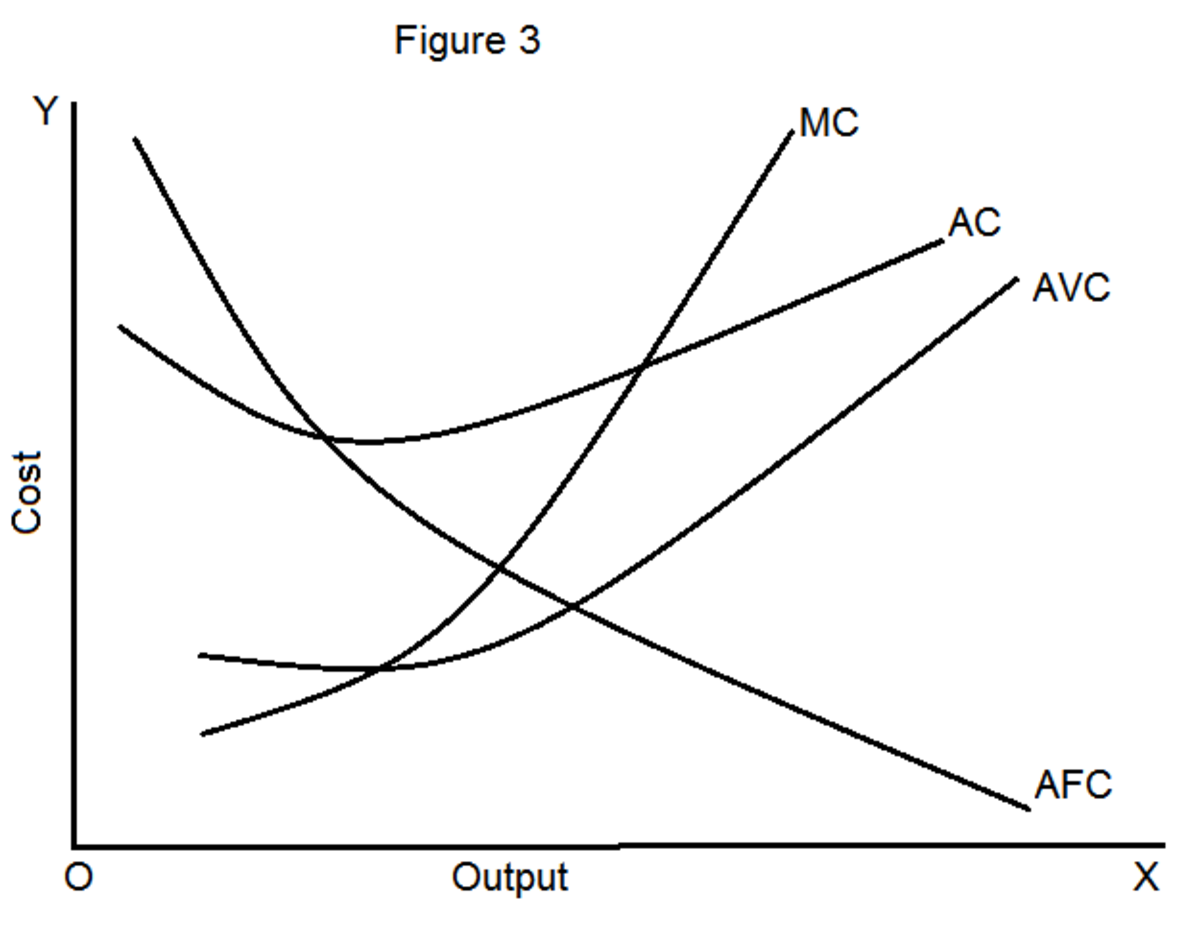Relationship between Average and Marginal Cost Curves

According to Prof. Richard Lipsey, the relationship between the marginal and the average cost is more a mathematical one rather than economics. The relationship can be briefly stated as follows:

1. When marginal cost is less than average cost, average cost is falling.

2. When marginal cost is greater than average cost, average cost is rising.

3. The MC curve must cut the AC curve at AC’s minimum point and from below.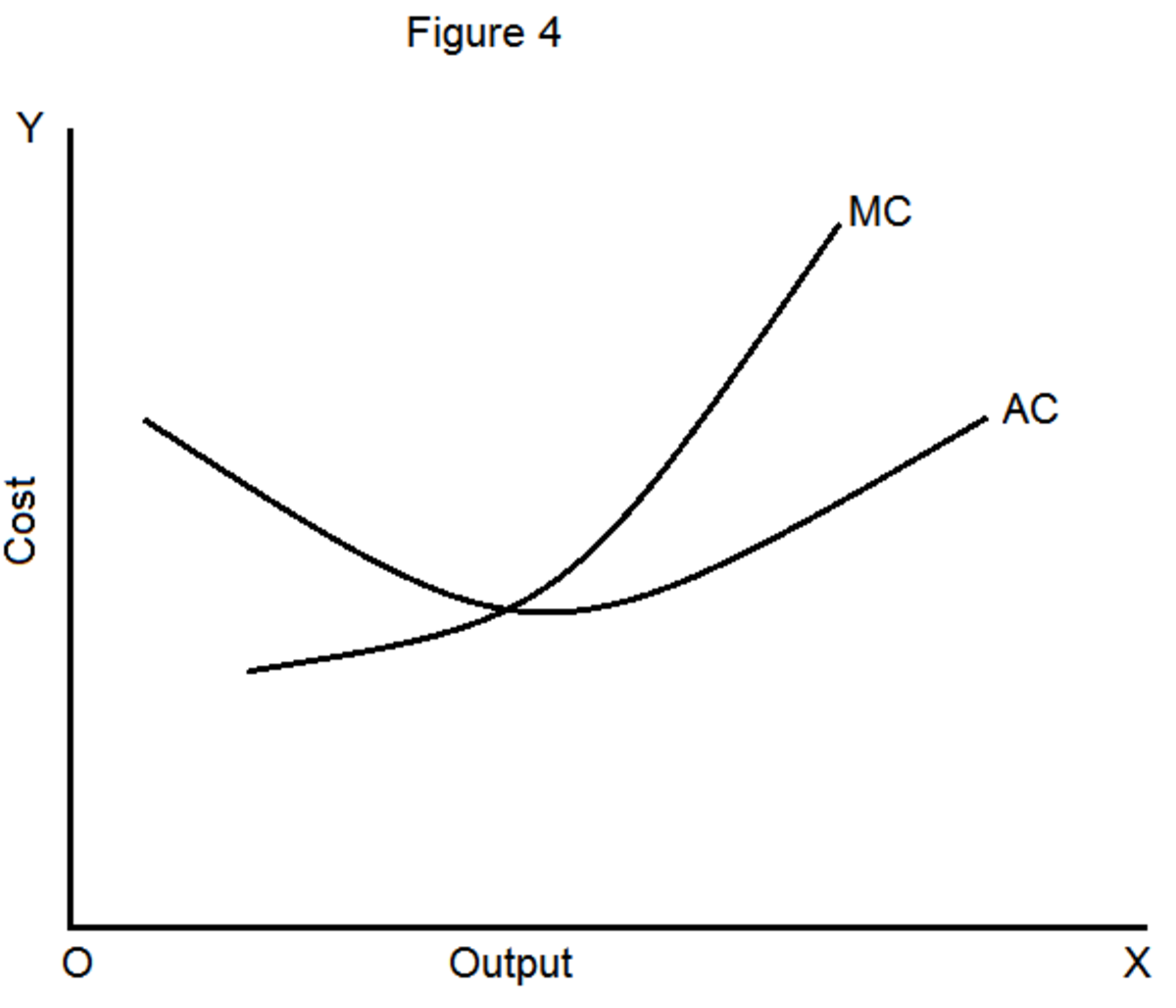These relationships can be easily explained with the help of a simple illustration from a cricket match.

Suppose that Mr. X’s batting average is 60. If in his next innings, he scores less than 60, say 56, his average will fall because his marginal score is less than his average score. If in the next innings, he scores more than 60, say 68, his average score will rise because his marginal score is greater than his average score. If, with the present average of 60, in the next innings also he scores just 60, then the average and marginal scores will be equal and his average score will neither rise nor fall.

This relationship can be easily illustrated with the help of the following chart: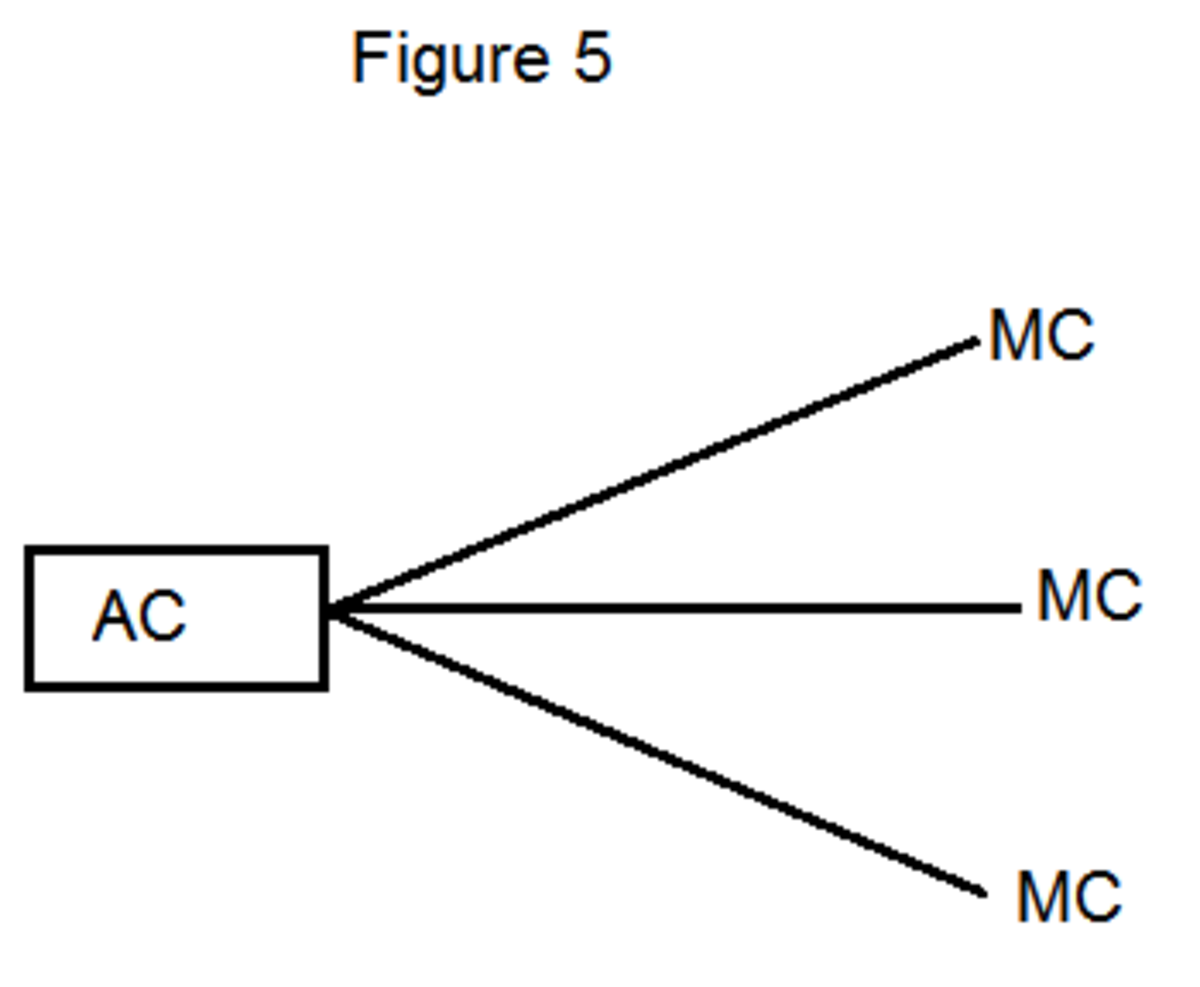When marginal cost is able the average cost, the AC rises because the MC pulls AC upwards. When marginal cost is below the average cost, the AC falls because the MC pulls AC downwards and when MC is the same as the AC, the average cost remains the same.